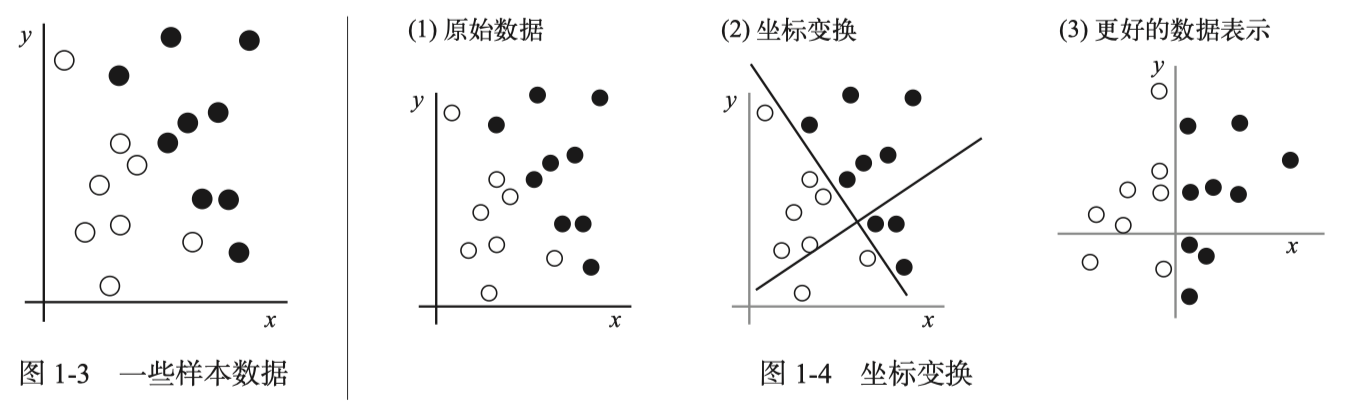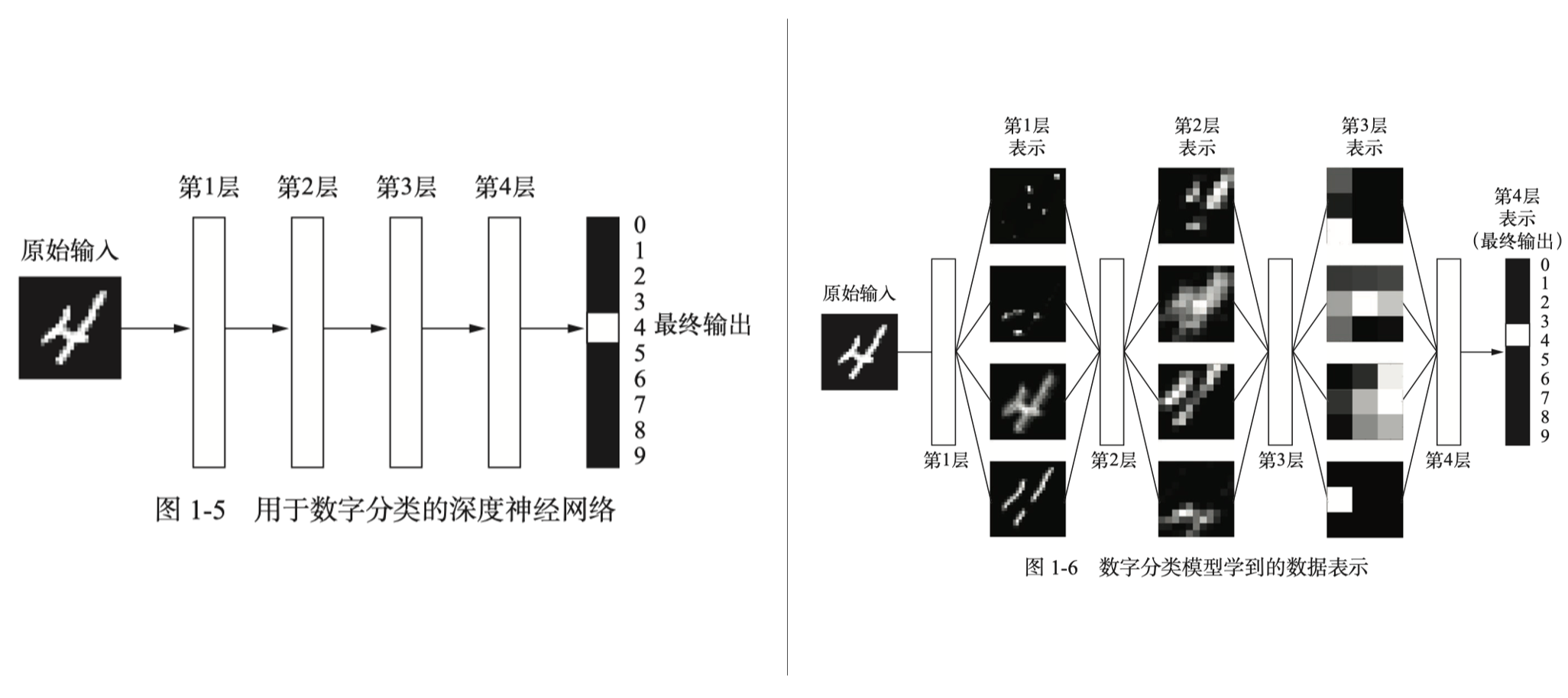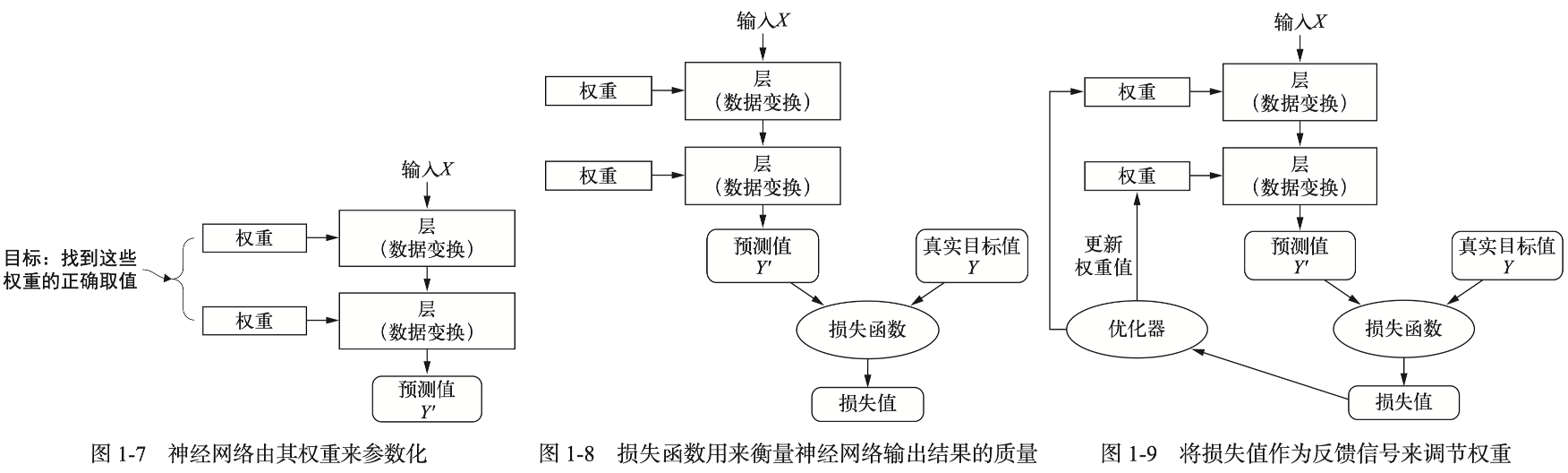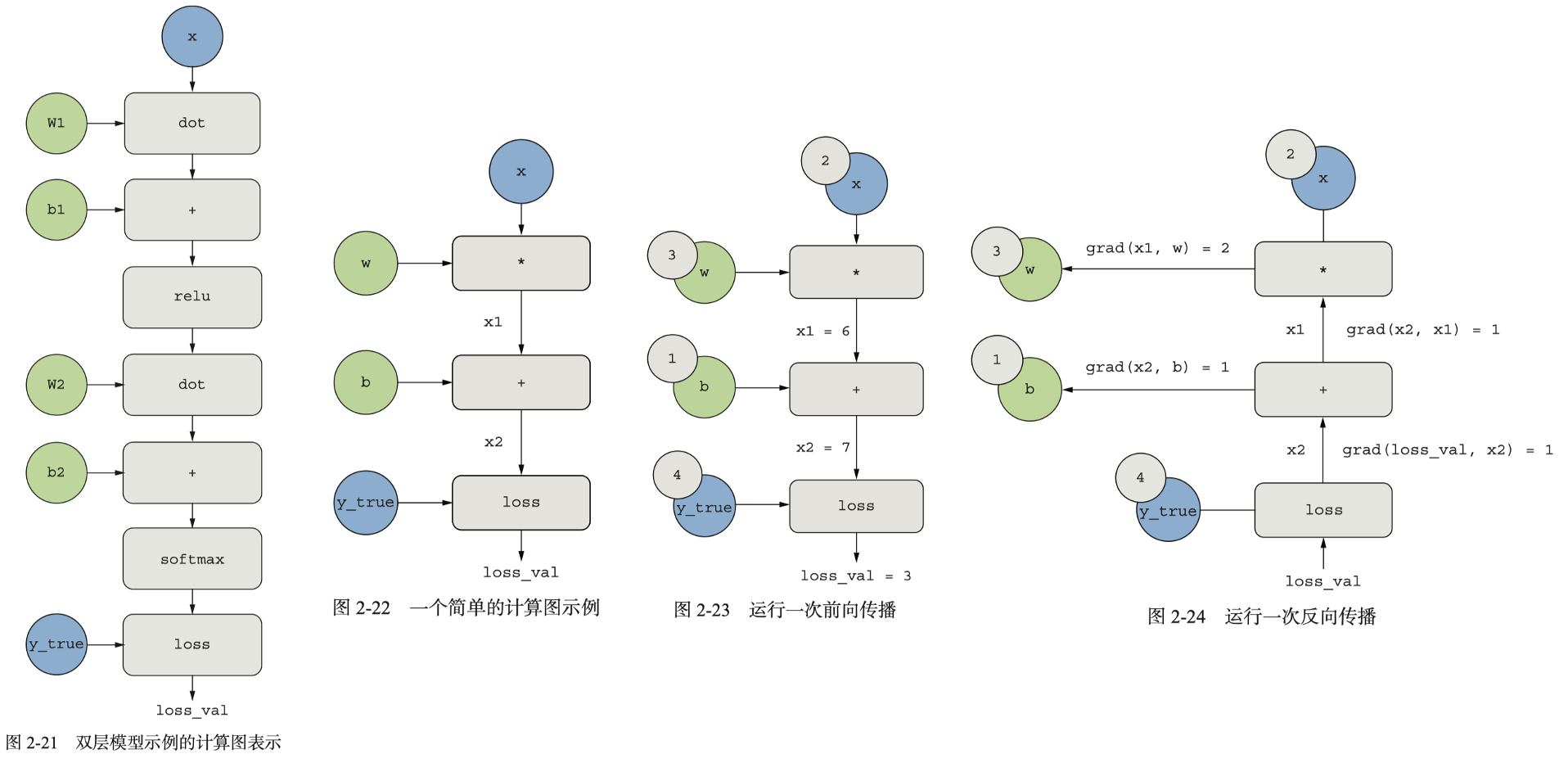## 1. 什么是深度学习

### 1.1 机器学习

graph LR; A("规则")-->B("经典程序设计"); C("数据")-->B; B-->D("答案");

graph LR; A("数据")-->B("机器学习"); C("答案")-->B; B-->D("规则");### 1.2 深度学习1. 通过逐层渐进的方式形成越来越复杂的表示。
2. 对中间的渐进表示共同进行学习，每一层的修改都需要同时考虑上下两层。

• 更好的神经层激活函数（activation funciton）
• 更好的权重初始化方案（weight-initialization scheme）

## 2. 神经网络的数学基础

### 2.1 Hello-World：手写数字分类任务

``````>>> from tensorflow.keras.datasets import mnist
>>> (train_images, train_labels), (test_images, test_labels) = mnist.load_data()
>>> train_images.shape
(60000, 28, 28)
>>> len(train_labels)
60000
>>> train_labels
array([5, 0, 4, ..., 5, 6, 8], dtype=uint8)
>>> test_images.shape
(10000, 28, 28)
>>> len(test_labels)
10000
>>> test_labels
array([7, 2, 1, ..., 4, 5, 6], dtype=uint8)
``````

``````from tensorflow import keras
from tensorflow.keras import layers
model = keras.Sequential([
layers.Dense(512, activation="relu"),
layers.Dense(10, activation="softmax")
])
model.compile(optimizer="rmsprop",
loss="sparse_categorical_crossentropy",
metrics=["accuracy"])
``````

`model.compile`用于为模型指定优化器（optimizer）、损失函数和指标（metric）——前者用于模型的自我更新（反向传播），中者用于衡量模型在训练数据上的性能，后者是我们想要在训练和测试过程中监控的模型性能。

``````train_images = train_images.reshape((60000, 28 * 28))
train_images = train_images.astype("float32") / 255
test_images = test_images.reshape((10000, 28 * 28))
test_images = test_images.astype("float32") / 255
``````

``````>>> model.fit(train_images, train_labels, epochs=5, batch_size=128)
Epoch 1/5
469/469 [==============================] - 3s 6ms/step - loss: 0.2677 - accuracy: 0.9234
Epoch 2/5
469/469 [==============================] - 3s 5ms/step - loss: 0.1086 - accuracy: 0.9672
Epoch 3/5
469/469 [==============================] - 3s 5ms/step - loss: 0.0714 - accuracy: 0.9790
Epoch 4/5
469/469 [==============================] - 3s 5ms/step - loss: 0.0511 - accuracy: 0.9848
Epoch 5/5
469/469 [==============================] - 3s 6ms/step - loss: 0.0379 - accuracy: 0.9883
<keras.callbacks.History object at 0x126416d60>
``````

``````>>> test_digits = test_images[0:10]
>>> predictions = model.predict(test_digits)
1/1 [==============================] - 0s 89ms/step
>>> predictions
array([8.9595602e-09, 2.3669788e-10, 6.6512412e-06, 2.7392696e-05,
3.0452769e-11, 4.7035979e-08, 9.1966051e-13, 9.9996525e-01,
7.0086877e-08, 4.8436243e-07], dtype=float32)
>>> predictions.argmax()
7
>>> predictions
0.99996525
>>> test_labels
7
``````

``````>>> test_loss, test_acc = model.evaluate(test_images, test_labels)
313/313 [==============================] - 1s 2ms/step - loss: 0.0648 - accuracy: 0.9815
>>> print(f"test_acc: {test_acc}")
test_acc: 0.9815000295639038
``````

### 2.2 神经网络的数据表示：张量

``````>>> import numpy as np
>>> x = np.array(12)
>>> print(x.ndim, x.shape)
0 ()
>>> y = np.array([12, 13, 14])
>>> print(y.ndim, y.shape)
1 (3,)
>>> z = np.array([[12, 13, 14], [15, 16, 17]])
>>> print(z.ndim, z.shape)
2 (2, 3)
``````

``````>>> train_images.ndim
2
>>> train_images.shape
(60000, 784)
>>> train_images.dtype
dtype('float32')
``````

NumPy中选择张量中特定元素的方法是张量切片（tensor slicing）：

``````>>> my_slice = train_images[10:100, :]
>>> my_slice.shape
(90, 784)
``````

### 2.3 神经网络的“齿轮”：张量运算

``````keras.layers.Dense(512, activation="relu")
# is something like
output = relu(dot(input, W) + b)
``````

1. 向较小张量添加轴（广播轴），使其ndim与较大张量相同。
2. 将较小张量沿着新轴重复，使其形状与较大张量相同。

``````train_images = train_images.reshape((60000, 28 * 28))
``````

### 2.4 神经网络的“引擎”：基于梯度的优化

``````output = relu(dot(input, W) + b)
``````

1. 抽取训练样本x和目标y_true组成一个数据批量。
2. 在x上运行模型（前向传播，forward pass），得到预测值y_pred。
3. 计算模型在这批数据上的损失值，用于衡量y_pred和y_true的差距。
4. 更新模型所有权重，从而略微减小模型在这批数据上的损失值。

``````loss_value = f(W)
W1 = W0 - step * grad(f(W0), W0)
``````

1. 计算损失相对于模型参数的梯度（反向传播，backward pass）。
2. 将参数沿着梯度反方向移动一小步，如`W -= learning_rate * gradient`

``````loss_value = loss(y_true, softmax(dot(relu(dot(inputs, W1) + b1), W2) + b2))
````````````>>> import tensorflow as tf